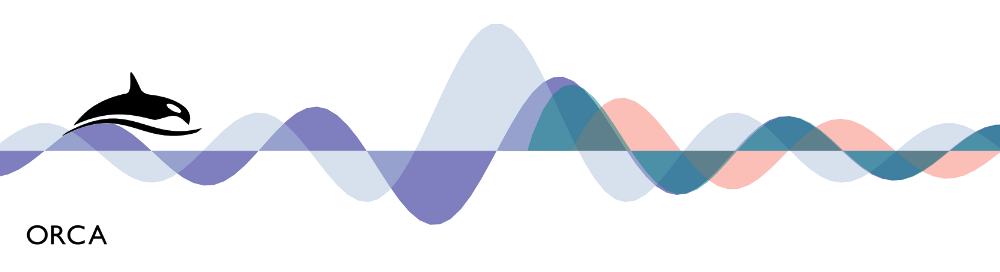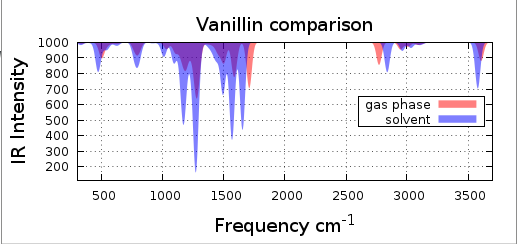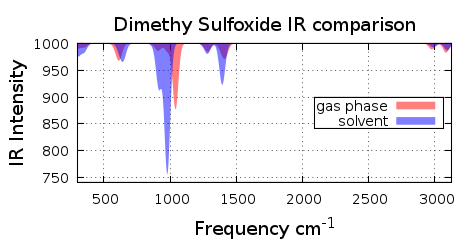HomeIndex

## 3 Solvent Effect

Gas phase calculation are very efficient and good in predicting properties of molecules with a low dipole moment. The majority of the molecules which we are interesting to study are in a solution. The calculated properties of molecules can vary, from slight changes to very different results, if we introduce the solvent effect.

To include this effect ORCA uses the conductor like screening model developed by Klamt. The solvent is represented as a dielectric polarizable continuum. In the COSMO aproach it is initially assumed  to be a perfect conductor which completey shields  (screens) the charge density of the solute.

## 3.1 Calculating with COSMO on ORCA

To calculate the solvent effect we just have to include the next line on the basic input

%cosmo epsilon #value
refrac  #value
end

Where epsilon is the dielectric constant and refrac is the refractive index of the solvent.

## 3.2  Infrared Specrum with and without Solvent

Lets se how properties vary with the solvent effect.

• Build the vanillin molecule of figure 3.2.1
• File input must look like this: vanillin.inp
• Perform a geometry optimization and a frequency analisys
Solvent Effect
• Do it again but with DMSO solvent (add the cosmo instructions)
• For DMSO epsilon= 47.2 and refrac=1.479
• Input file must look like this: vanillin_solvent.inpFigure 3.2.1 Vanillin molecule

## 3.3 Visualize the Spectrums.

Plot both of the IR spectrums and notice the differences, figure 3.3.1.
As you can see in figure 3.3.1 there is a shift on the peaks.
Compare the spectrum with the experimental IR, you can see it here.
See the comparison on the HIRSHFELD charge here
Here you can find the ourputs:  vanillin_gas.out vanillin_solvent.out
.Figure 3.3.1 Vanillin IR spectrum

## 3.4 DMSO with DMSO as solvent

See the comparison Spectra of DMSO with and without solvent in figure 3.4.1Figure 3.4.1 DMSO Spectra ComparisonLABFAMA